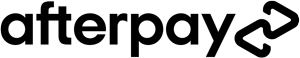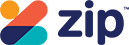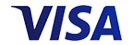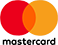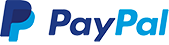Shop the Official Ecology 2021 Christmas Gift Guide! Guaranteed to bring joy and smiles no matter the price or the recipient! There's something for everyone here.WAS \$12.95 NOW \$9.95
Increase value Decrease value
WAS \$12.95 NOW \$9.95
Increase value Decrease value
WAS \$12.95 NOW \$9.95
Increase value Decrease value
\$29.95
Increase value Decrease value
\$49.95
Increase value Decrease value
\$59.95
Increase value Decrease value
\$14.95
Increase value Decrease value
\$14.95
Increase value Decrease value
WAS \$24.95 NOW \$19.95
Increase value Decrease value
WAS \$24.95 NOW \$19.95
Increase value Decrease value
\$12.95
Increase value Decrease value
\$12.95
\$12.95
\$12.95
\$39.95
Increase value Decrease value
\$19.95
Increase value Decrease value
\$34.95
Increase value Decrease value
WAS \$49.95 NOW \$37.46
Increase value Decrease valueWAS \$49.95 NOW \$24.95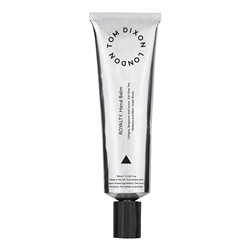\$65.00
Increase value Decrease value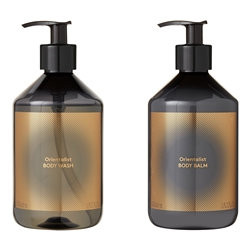\$150.00
Increase value Decrease value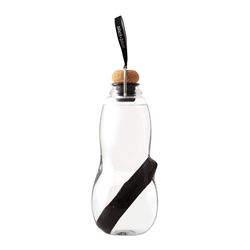WAS \$39.95 NOW \$29.95
Increase value Decrease value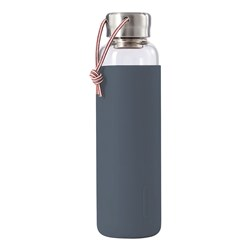WAS \$39.95 NOW \$29.95
Increase value Decrease value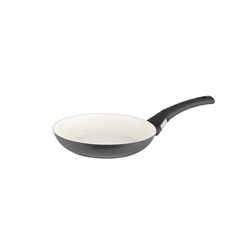WAS \$89.95 NOW \$49.95
Increase value Decrease value
\$29.95
Increase value Decrease value
\$39.95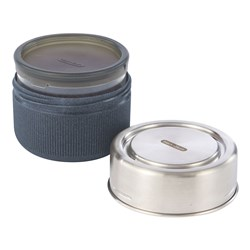WAS \$44.95 NOW \$29.95
Increase value Decrease value
WAS \$69.95 NOW \$59.95
Increase value Decrease value
WAS \$9.95 NOW \$7.95
Increase value Decrease value
\$49.95
Increase value Decrease value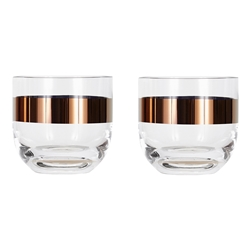\$125.00
Increase value Decrease value
\$39.95
Increase value Decrease value
\$39.95
Increase value Decrease value
WAS \$49.95 NOW \$34.97
Increase value Decrease value
\$39.95
Increase value Decrease value
\$49.95
Increase value Decrease value
\$49.95
Increase value Decrease value\$70.00
Increase value Decrease value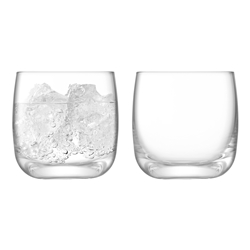\$64.95
Increase value Decrease value\$79.95
Increase value Decrease value\$29.95
Increase value Decrease value
\$29.95
Increase value Decrease value
WAS \$12.95 NOW \$9.95
Increase value Decrease value
WAS \$12.95 NOW \$9.95
Increase value Decrease value
WAS \$12.95 NOW \$9.95
Increase value Decrease value
WAS \$12.95 NOW \$9.95
Increase value Decrease value
WAS \$12.95 NOW \$9.95
Increase value Decrease value
\$29.95
Increase value Decrease value
\$29.95
\$14.95
Increase value Decrease value
\$14.95
Increase value Decrease value
WAS \$24.95 NOW \$19.95
Increase value Decrease value
\$12.95
\$12.95
Increase value Decrease value
\$12.95
\$12.95
\$12.95
Increase value Decrease value
\$12.95
\$19.95
Increase value Decrease value
\$19.95
Increase value Decrease value
\$29.95
Increase value Decrease value
\$24.95
Increase value Decrease value
WAS \$19.95 NOW \$14.96
Increase value Decrease valueWAS \$49.95 NOW \$24.95\$59.95
Increase value Decrease value
\$159.95
Increase value Decrease value
\$139.95
Increase value Decrease value
\$29.95
Increase value Decrease value
\$49.95
Increase value Decrease value
\$49.95
Increase value Decrease value
\$49.95
Increase value Decrease value
\$79.95
Increase value Decrease value
\$59.95
Increase value Decrease value
\$79.95
Increase value Decrease value
\$99.95
Increase value Decrease value
\$69.95
Increase value Decrease value
\$49.95
Increase value Decrease value
\$39.95
Increase value Decrease value
\$69.95
\$59.95
Increase value Decrease value
\$129.95
Increase value Decrease value
\$169.95
Increase value Decrease value
\$59.95
Increase value Decrease value
\$44.95
Increase value Decrease value\$94.95
Increase value Decrease value\$79.95
Increase value Decrease value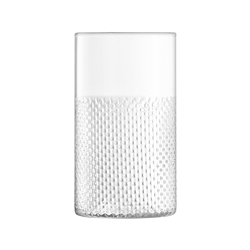\$94.95
Increase value Decrease value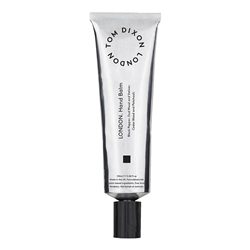\$65.00
Increase value Decrease value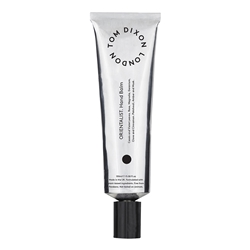\$65.00
Increase value Decrease value\$95.00
Increase value Decrease value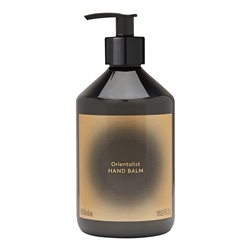\$95.00
Increase value Decrease value\$29.95
Increase value Decrease value
\$29.95
Increase value Decrease value
WAS \$12.95 NOW \$9.95
Increase value Decrease value
WAS \$12.95 NOW \$9.95
Increase value Decrease value
WAS \$12.95 NOW \$9.95
Increase value Decrease value
WAS \$12.95 NOW \$9.95
Increase value Decrease value
WAS \$12.95 NOW \$9.95
Increase value Decrease value
\$29.95
Increase value Decrease value
\$29.95
\$14.95
Increase value Decrease value
\$14.95
Increase value Decrease value
WAS \$24.95 NOW \$19.95
Increase value Decrease value
\$12.95
\$12.95
Increase value Decrease value
\$12.95
\$12.95
\$12.95
Increase value Decrease value
\$12.95
\$19.95
Increase value Decrease value
\$19.95
Increase value Decrease value
\$29.95
Increase value Decrease value
\$24.95
Increase value Decrease value
WAS \$19.95 NOW \$14.96
Increase value Decrease valueWAS \$49.95 NOW \$24.95\$49.95
Increase value Decrease value
\$49.95
Increase value Decrease value
\$39.95
Increase value Decrease value
\$49.95
Increase value Decrease value
\$49.95
\$49.95
Increase value Decrease valueWAS \$39.95 NOW \$29.95
Increase value Decrease valueWAS \$39.95 NOW \$29.95
Increase value Decrease valueWAS \$89.95 NOW \$49.95
Increase value Decrease value
\$49.95
Increase value Decrease value
\$49.95
Increase value Decrease value
\$39.95
Increase value Decrease value
\$49.95
Increase value Decrease value
\$49.95
Increase value Decrease value
\$44.95
Increase value Decrease value
\$34.95
Increase value Decrease value
\$49.95
Increase value Decrease value
\$39.95
Increase value Decrease value
\$39.95
Increase value Decrease value
\$49.95
Increase value Decrease value
\$34.95
Increase value Decrease value
WAS \$49.95 NOW \$37.46
Increase value Decrease valueWAS \$69.95 NOW \$34.95
Increase value Decrease value\$59.95
Increase value Decrease value
\$139.95
Increase value Decrease value
\$79.95
Increase value Decrease value
\$59.95
Increase value Decrease value
\$79.95
Increase value Decrease value
\$99.95
Increase value Decrease value
\$69.95
Increase value Decrease value
\$59.95
Increase value Decrease value
\$69.95
\$59.95
Increase value Decrease value
\$59.95
Increase value Decrease value\$94.95
Increase value Decrease value\$79.95
Increase value Decrease value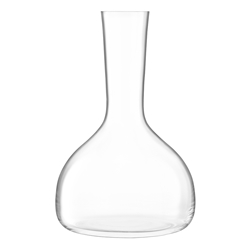\$79.95
Increase value Decrease value\$94.95
Increase value Decrease value\$65.00
Increase value Decrease value\$65.00
Increase value Decrease value\$95.00
Increase value Decrease value\$95.00
Increase value Decrease value\$179.95
Increase value Decrease value
\$159.95
Increase value Decrease value
\$159.95
Increase value Decrease value\$179.95
Increase value Decrease value
\$159.95
Increase value Decrease value
\$169.95
Increase value Decrease value
\$159.95
Increase value Decrease value\$179.95
Increase value Decrease value
\$139.95
Increase value Decrease value
\$129.95
Increase value Decrease value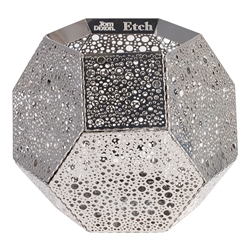\$110.00
Increase value Decrease value\$104.95
Increase value Decrease value
\$129.95
Increase value Decrease value
\$129.95
Increase value Decrease value
\$129.95
Increase value Decrease value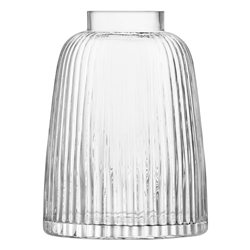\$124.95
Increase value Decrease value
\$169.95
Increase value Decrease value
\$169.95
Increase value Decrease value
\$169.95
Increase value Decrease value
WAS \$129.95 NOW \$89.95
Increase value Decrease value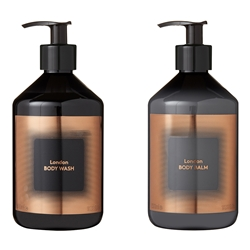\$150.00
Increase value Decrease value
\$189.95
Increase value Decrease value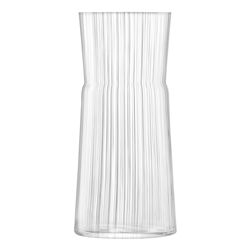\$179.95
Increase value Decrease value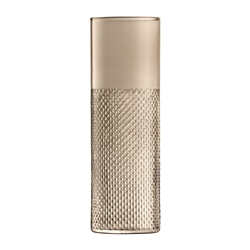\$159.95
Increase value Decrease value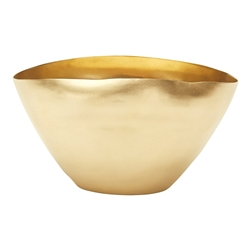\$150.00
Increase value Decrease value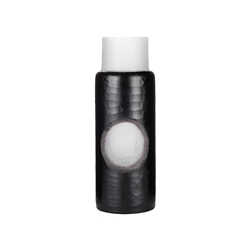\$190.00
Increase value Decrease value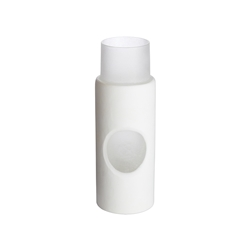\$190.00
Increase value Decrease value\$150.00
Increase value Decrease value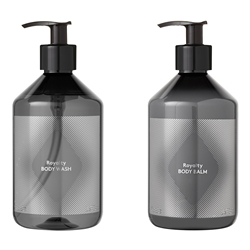\$150.00
Increase value Decrease value